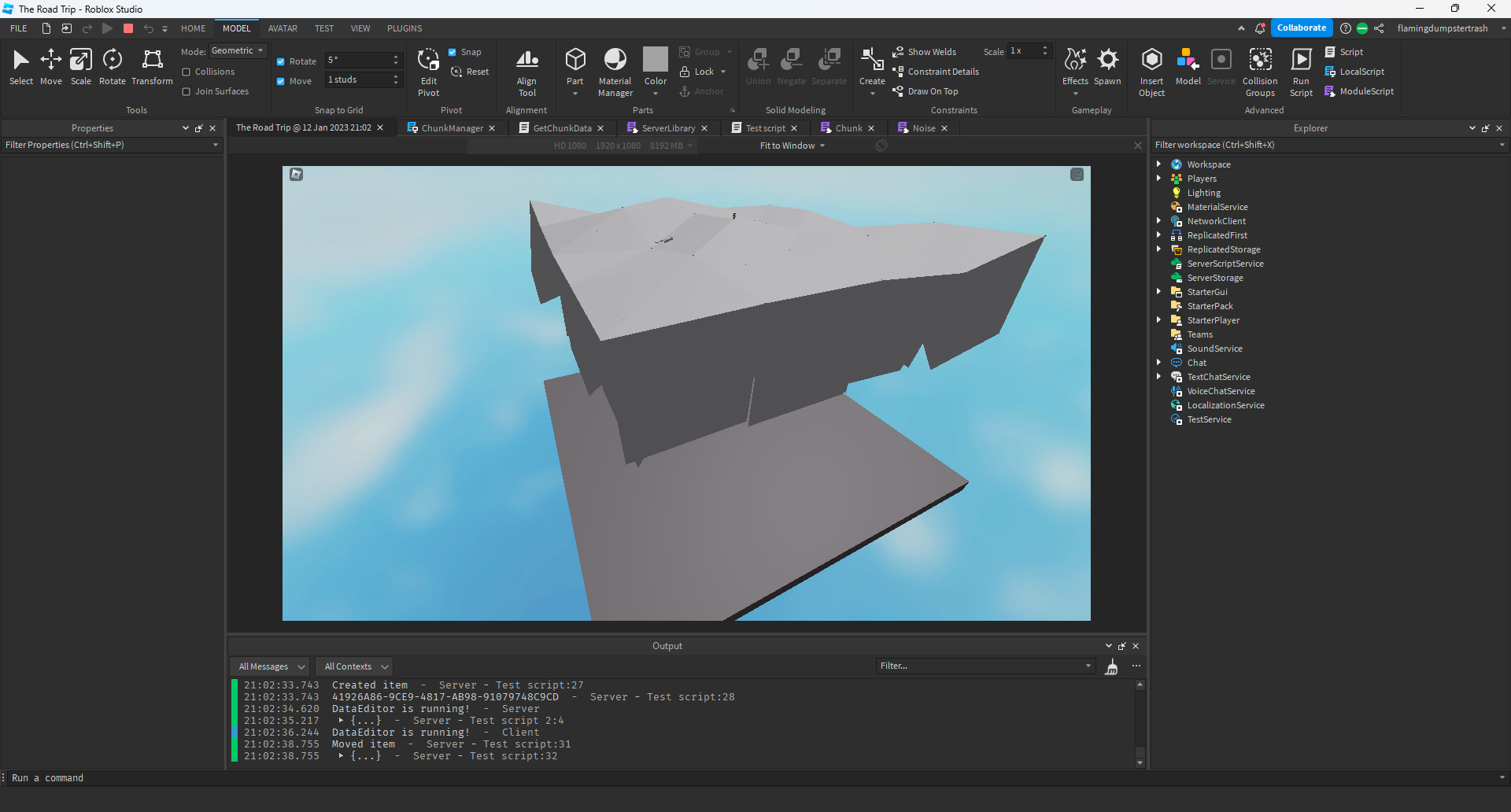# Resizing a part from a single side at an angle

1. What do you want to achieve?
I’m trying to make terrain generation similar to in this game Road to Gramby's 👵 - Roblox
as you can see the terrain looks good and the parts are not super thin (you can see this in the second picture)

2. What is the issue?

when doing this myself, the parts are not lined up correctly.

3. What solutions have you tried so far?
i have tried using BasePart:Resize which only ever returns false and does nothing for me. i have also tried changing the cframe to adjust for the resize, but this does not work either and i think this is due to the part being angled.

this is the effect i want to achieve. i did this by using the resize tool, but i want to be able to do it in code.

this is the code I currently use to create triangles:

``````local wedge = Instance.new("WedgePart");
wedge.Anchored = true;
wedge.TopSurface = Enum.SurfaceType.Smooth;
wedge.BottomSurface = Enum.SurfaceType.Smooth;

local thickness = 0

function Functions.DrawTriangle(a,b,c,parent)
local ab, ac, bc = b - a, c - a, c - b;
local abd, acd, bcd = ab:Dot(ab), ac:Dot(ac), bc:Dot(bc);

if (abd > acd and abd > bcd) then
c, a = a, c;
elseif (acd > bcd and acd > abd) then
a, b = b, a;
end

ab, ac, bc = b - a, c - a, c - b;

local right = ac:Cross(ab).unit;
local up = bc:Cross(right).unit;
local back = bc.unit;

local height = math.abs(ab:Dot(up));

local w1 = wedge:Clone();
w1.Size = Vector3.new(thickness, height, math.abs(ab:Dot(back)));
w1.CFrame = CFrame.fromMatrix((a + b)/2, right, up, back);
w1.Parent = parent;

local w2 = wedge:Clone();
w2.Size = Vector3.new(thickness, height, math.abs(ac:Dot(back)));
w2.CFrame = CFrame.fromMatrix((a + c)/2, -right, up, -back);
w2.Parent = parent;

return w1, w2;
end
``````

If youre looking for a solution, making these changes fixed it for me:

``````wedge.Anchored = true;
wedge.TopSurface = Enum.SurfaceType.Smooth;
wedge.BottomSurface = Enum.SurfaceType.Smooth;

local thickness = 100

function Functions.DrawTriangle(a,b,c,parent)
local ab, ac, bc = b - a, c - a, c - b;
local abd, acd, bcd = ab:Dot(ab), ac:Dot(ac), bc:Dot(bc);

if (abd > acd and abd > bcd) then
c, a = a, c;
elseif (acd > bcd and acd > abd) then
a, b = b, a;
end

ab, ac, bc = b - a, c - a, c - b;

local right = ac:Cross(ab).unit;
local up = bc:Cross(right).unit;
local back = bc.unit;

local height = math.abs(ab:Dot(up));

local w1 = wedge:Clone();
w1.Size = Vector3.new(thickness, height, math.abs(ab:Dot(back)));
w1.CFrame = CFrame.fromMatrix((a + b)/2, right, up, back);
w1.Parent = parent;

if w1.CFrame.RightVector.Y == math.abs(w1.CFrame.RightVector.Y) then
w1.CFrame += -w1.CFrame.RightVector * thickness/2
else
w1.CFrame += w1.CFrame.RightVector * thickness/2
end

local w2 = wedge:Clone();
w2.Size = Vector3.new(thickness, height, math.abs(ac:Dot(back)));
w2.CFrame = CFrame.fromMatrix((a + c)/2, -right, up, -back);
w2.Parent = parent;

if w2.CFrame.RightVector.Y == math.abs(w2.CFrame.RightVector.Y) then
w2.CFrame -= w2.CFrame.RightVector * thickness/2
else
w2.CFrame -= -w2.CFrame.RightVector * thickness/2
end

return w1, w2;
end
``````This topic was automatically closed 14 days after the last reply. New replies are no longer allowed.